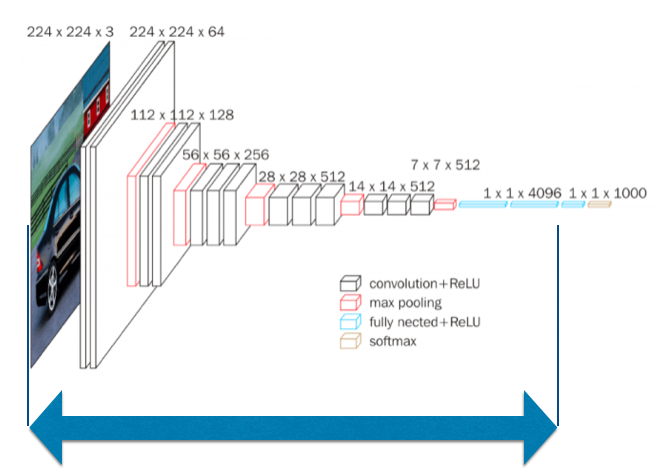# Computing Image Similarity with pre-trained Keras models

Source: Deep Learning on Medium# Computing Image Similarity with pre-trained Keras models

There are many pre-trained image classification deep learning models available in Keras and Tensorflow libraries; for example, ImageNet, VGG16, ResNet, and etc. These models consist of blocks of convolutional neural networks followed by fully connected layers for final classification. These models can be fine-tuned for classifying different labels in specific use cases or used for feature extraction.

In this article, I used VGG16 for feature extracting geological images in order to compute image similarity between the images. I have provided sample data and code in my github repo.

First, the images need to be loaded, read and saved in an array.

`from keras.preprocessing.image import load_imgfrom keras.preprocessing.image import img_to_arrayimport osdef load_images_from_folder(folder): images = [] for filename in os.listdir(folder): img = load_img(os.path.join(folder,filename), target_size (224, 224))  img = img_to_array(img) img = img.reshape((1,) + img.shape) if img is not None: images.append(img) return images`

The second step is to load the model in order to extract the needed features from the images. In the load_image_from_folder(folder) function I am reshaping the images so that they are the same shape as the input of the VGG16.

First, the needed libraries are imported. The first function below loads the VGG16 model, and another model is created with all the weights except for the last 2 layers, then the model gets saved it in a given path. The second function reads the saved model.

`from keras.applications.vgg16 import VGG16from keras import models, Modeldef create_model(save_filepath): # loading vgg16 model and using all the layers until the 2 to the last to use all the learned cnn layers ssl._create_default_https_context = ssl._create_unverified_context vgg = VGG16(include_top=True) model2 = Model(vgg.input, vgg.layers[-2].output) model2.save(save_filepath) # saving the model just in case return model2def load_model_from_path(save_filepath): # loading the model from the saved path model = load_model(save_filepath) return model`

Now, it’s time for feature extraction, predicting from the created model.

`def get_preds(all_imgs_arr,model): # getting the extracted features - final shape (number_of_images, 4096) preds = model.predict(all_imgs_arr) return preds`

The extracted feature vectors can be then used to compute image similarity using Annoy.

`def get_nearest_neighbor_and_similarity(preds, K): dims = 4096 n_nearest_neighbors = K+1 trees = 10000 file_index_to_file_vector = {}# build ann index t = AnnoyIndex(dims) for i in range(preds.shape):file_vector = preds[i] file_index_to_file_vector[i] = file_vector t.add_item(i, file_vector) t.build(trees)for i in range(preds.shape): master_vector = file_index_to_file_vector[i]named_nearest_neighbors = [] similarities = [] nearest_neighbors = t.get_nns_by_item(i, n_nearest_neighbors) for j in nearest_neighbors:# print (j) neighbor_vector = preds[j] similarity = 1 - spatial.distance.cosine(master_vector, neighbor_vector) rounded_similarity = int((similarity * 10000)) / 10000.0 similarities.append(rounded_similarity) return similarities, nearest_neighborsdef get_similar_images(similarities, nearest_neighbors, images1): j = 0 for i in nearest_neighbors: show_img(images1[i]) print (similarities[j]) j+=1`

Here is an example of the results:

Here is a blog that helped me: https://douglasduhaime.com/posts/identifying-similar-images-with-tensorflow.html